Courses

# Impulse Invariance Method Electrical Engineering (EE) Notes | EduRev

## Electrical Engineering (EE) : Impulse Invariance Method Electrical Engineering (EE) Notes | EduRev

The document Impulse Invariance Method Electrical Engineering (EE) Notes | EduRev is a part of the Electrical Engineering (EE) Course Digital Signal Processing.
All you need of Electrical Engineering (EE) at this link: Electrical Engineering (EE)

Impulse Invariance Method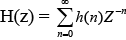H(z) (at z =e ST ) = ∑h(n)e - STn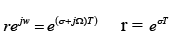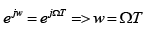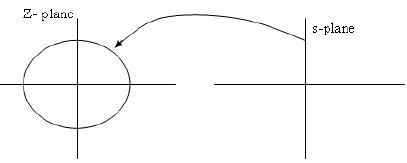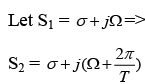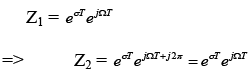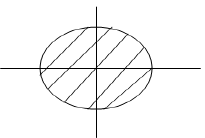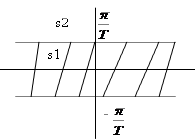If the real part is same, imaginary part is differ by integral multiple of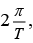this is the biggest disadvantage of Impulse Invariance method.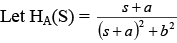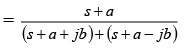hA(t) =e-at  Cosbt    for t ≥ 0          s1 = -a-jb

= 0        otherwise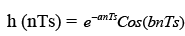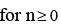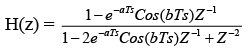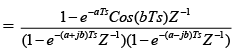The pole located at s=p is transformed into a pole in the Z-plane at Z = e PTS, however, the finite zero located in the s-plane at s= -a was not converted into a zero in the z-plane at Z = e-aTs , although the zero at s=∞ was placed at z=0.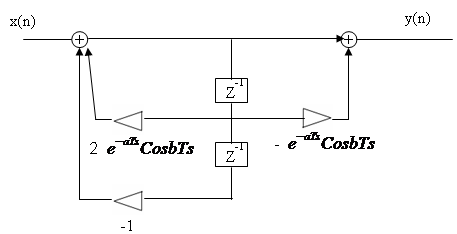Desing a Chebyshev LPF using Impulse-Invariance Method.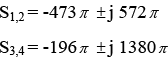[The freq response for analog filter we plotted over freq range 0 to 10000 π. To set the discrete-time freq range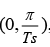, therefore Ts =10-4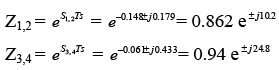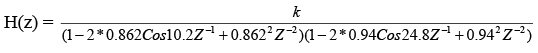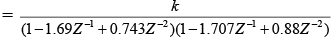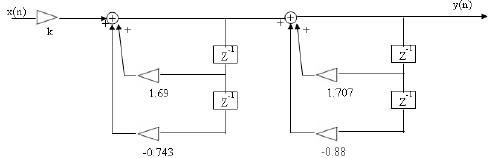Methods to convert analog filters into Digital filters:

1. By approximation of derivatives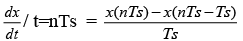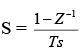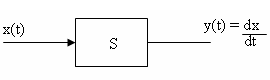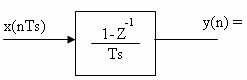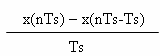Or

Using forward-difference mapping based on first order approximation Z = e sTs≌ 1+STs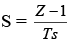Using backward- difference mapping is based on first order approximation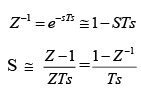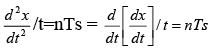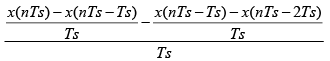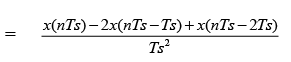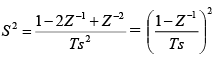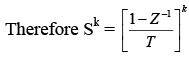Therefore H(z) =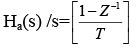using backward difference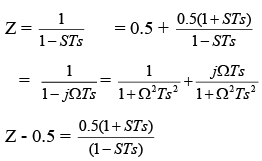lz - 0.5| = 0.5 is mapped into a circle of radius 0.5, centered at Z=0.5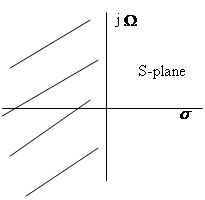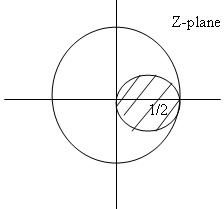Using Forward-difference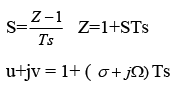if σ =0 u=1 and j Ω axis maps to Z=1

If σ >0, then u>1, the RHS-plane maps to right of z=1.

If σ <0, then u<1, the LHS-plane maps to left of z=1.

The stable analog filter may be unstable digital filter.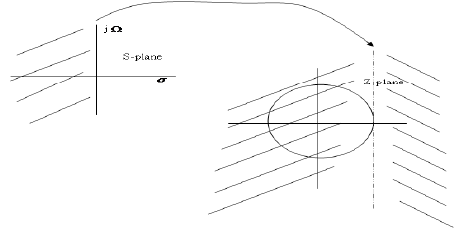Bilinear Transformation

• Provides a non linear one to one mapping of the frequency points on the jw axis in s -plane to those on the unit circle in the z-plane.
• This procedure also allows us to implement digital HP filters from their analog
counter parts.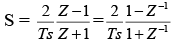{Using trapezoidal rule y(n)=y(n-1)+0.5Ts[x(n)+x(n-1)]

H(Z)=2(Z-1) / [Ts(Z+1)]    }

To find H(z), each occurrence of S in HA(s) is replaced by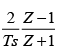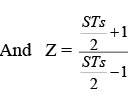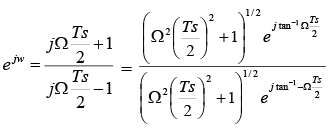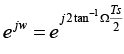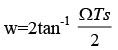The entire j Ω axis in the s-plane - ∞ <j Ω<∞ maps exactly once onto the unit circle - π< ≤ π  such that there is a one to one correspondence between the continuous -time and discrete time frequency points. It is this one to one mapping that allows analog HPF to be implemented in digital filter form.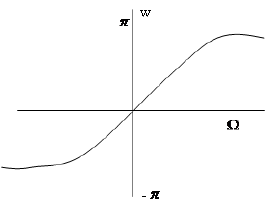As in the impulse invariance method, the left half of s-plane maps on to the inside of the unit circle in the z-plane and the right half of s-plane maps onto the outside.

In Inverse relationship is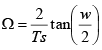For smaller value of frequency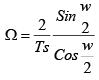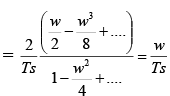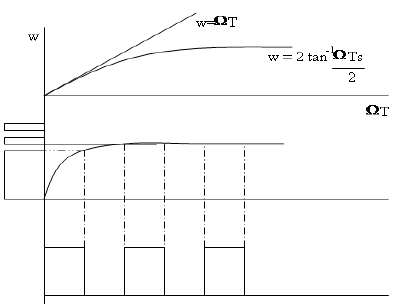(B.W of higher freq pass band will tend to reduce disproportionately)

The mapping is ≌ linear for small Ω  and w. For larger freq values, the non linear compression that occurs in the mapping of Ω to w is more apparent. This compression causes the transfer function at the high Ω freq to be highly distorted when it is translated to
the w-domain.
Prewarping Procedure:

When the desired magnitude response is piece wise constant over frequency, this compression can be compensated by introducing a suitable prescaling or prewarping to the Ω  freq scale. Ω  scale is converted into Ω * scale.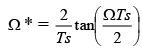We now derive the rule by which the poles are mapped from the s-plane to the z-plane.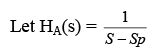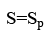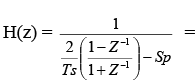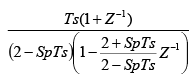A pole at S=Sp in the s-plane gets mapped into a zero at z= -1 and a pole at Z =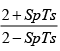Ex:

Chebyshev LPF design using the Bilinear Transformation

Pass band:

-1<|H ( jΩ)|dB≤0   for  0 ≤ Ω ≤ 1404π=4411 rad

Stop band:

|H ( jΩ)| dB < -60 for Ω ≥ 8268 π rad/sec  =25975 rad/s

Let the Ts = 10-4 sec

Prewarping values are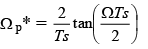= 2*104 tan(0.0702π ) = 4484 rad/sec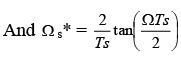= 2*104 tan(0.4134π ) = 71690 rad/sec

The modified specifications are

Pass band:

-1<lH ( jΩ*)|dB≤ 0 for  0 ≤ Ω * ≤  4484 rad/s

Stop band:

|H ( jΩ*)| dB < - 60   for Ω *≥ 71690rad/sec

Value of μ : is determined from the pass band ripple    10log  (1 + m -2 ) -1 > -1dB

μ= 0.508

Value of N: is determined from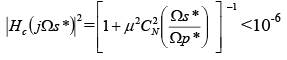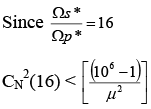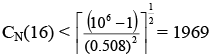C3(16) = 16301

N = 3 is sufficient

Using Impulse Invariance method a value of N=4 was required.

ρ=4.17

Major  R  =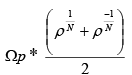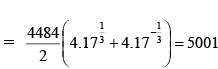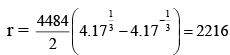Since there are three poles, the angles are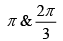S1 = r cosθ + j Rsinθ = -2216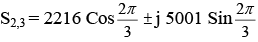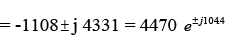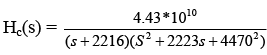Pole Mapping

At S=S1

In the Z-plane there is zero at Z = -1 and pole at Z =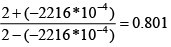S2,3 = there are two zeros at Z=-1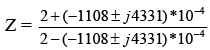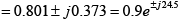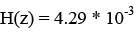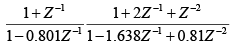Pole Mapping Rules:

Hz(z) = 1-CZ-1 zero at Z=C and pole at Z = 0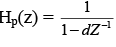pole ar Z=d and zero at z=0

C and d can be complex-valued number.

Pole Mapping for Low-Pass to Low Pass Filters

Applying low pass to low pass transformation to Hz(z) α we get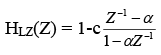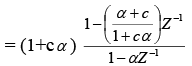The low pass zero at z=c is transformed into a zero at z=C1 where C1 =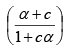And pole at z=0 is Z=α

Similarly,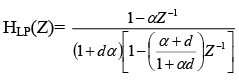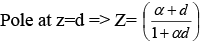Zero at z=0 => z =α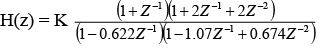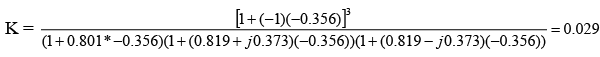Offer running on EduRev: Apply code STAYHOME200 to get INR 200 off on our premium plan EduRev Infinity!

,

,

,

,

,

,

,

,

,

,

,

,

,

,

,

,

,

,

,

,

,

;